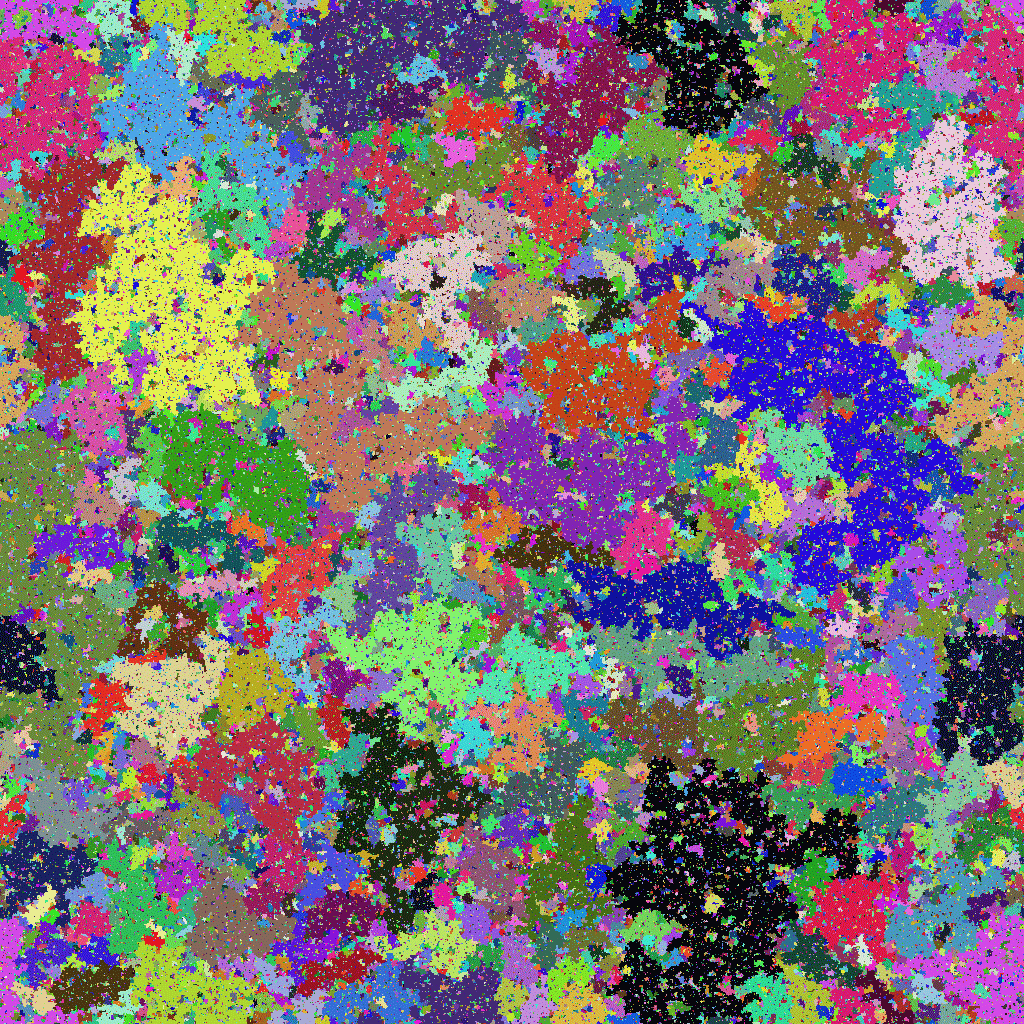# Leaps in long-range connectivity

Advancing the mathematical theory of bootstrap percolation, where active cells on a lattice with few active neighbours cease to be active.

Bootstrap percolation originated as a simple cellular automata model of the spread of infection. Given a set of initially infected sites, in consecutive rounds sites become infected if they satisfy a certain infection rule, for example, more than a given number of their neighbours are infected. Percolation occurs if every node is eventually infected.

In this project we investigate the typical and extremal properties of bootstrap percolation for various infection rules and underlying networks. For sites that are initially randomly infected, we study the critical density of infection that leads to percolation. Alternatively, if the initial infected sites are set by hand, we study how many infected nodes are necessary to infect the whole network.

Bootstrap percolation can be used to model many real-life phenomena, such as pandemics, computer network failure, ferromagnetic behaviour, the spread of voting preferences and information processing in neural networks. A better mathematical theory will help us understand these processes and draw reliable conclusions well beyond what can be achieved through computer simulations.## Papers in this project

•Journal of Statistical Mechanics

### Clusters of neurons

Percolation theory shows that the formation of giant clusters of neurons relies on a few parameters that could be measured experimentally.

•Transactions of the American Mathematical Society

### Bootstrap percolation models

A subset of bootstrap percolation models, which stabilise systems of cells on infinite lattices, exhibit non-trivial phase transitions.

•SIAM Journal on Discrete Mathematics

### Maximum percolation time

A simple formula gives the maximum time for an n x n grid to become entirely infected having undergone a bootstrap percolation process.

•Electronic Journal of Probability

### Percolation on Galton-Watson trees

The critical probability for bootstrap percolation, a process which mimics the spread of an infection in a graph, is bounded for Galton-Watson trees.